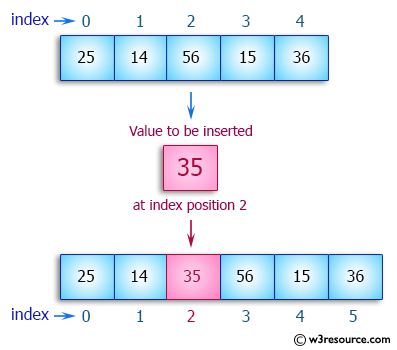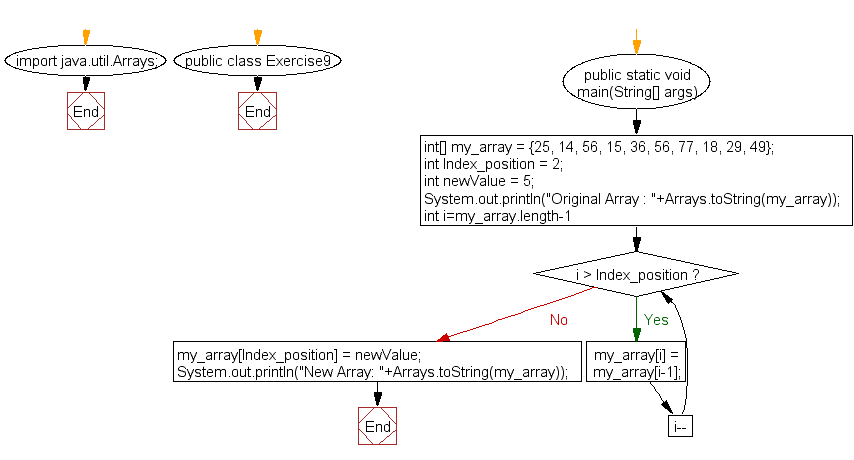﻿ Java exercises: Insert an element into an array - w3resource# Java Array Exercises: Insert an element into an array

## Java Array: Exercise-9 with Solution

Write a Java program to insert an element (specific position) into an array.

Pictorial Presentation:Sample Solution:

Java Code:

``````import java.util.Arrays;
public class Exercise9 {

public static void main(String[] args) {

int[] my_array = {25, 14, 56, 15, 36, 56, 77, 18, 29, 49};

// Insert an element in 3rd position of the array (index->2, value->5)

int Index_position = 2;
int newValue    = 5;

System.out.println("Original Array : "+Arrays.toString(my_array));

for(int i=my_array.length-1; i > Index_position; i--){
my_array[i] = my_array[i-1];
}
my_array[Index_position] = newValue;
System.out.println("New Array: "+Arrays.toString(my_array));
}
}
```
```

Sample Output:

```Original Array : [25, 14, 56, 15, 36, 56, 77, 18, 29, 49]
New Array: [25, 14, 5, 56, 15, 36, 56, 77, 18, 29]
```

Flowchart:Visualize Java code execution (Python Tutor):

Java Code Editor:

Improve this sample solution and post your code through Disqus

What is the difficulty level of this exercise?

Test your Programming skills with w3resource's quiz.

﻿

## Java: Tips of the Day

countOccurrences

Counts the occurrences of a value in an array.

Use Arrays.stream().filter().count() to count total number of values that equals the specified value.

```public static long countOccurrences(int[] numbers, int value) {
return Arrays.stream(numbers)
.filter(number -> number == value)
.count();
}
```

Ref: https://bit.ly/3kCAgLb